## Fluid Flow

When a fluid is viewed on a molecular, that is, on a small, scale, the properties of the fluid have an extremely nonuniform spatial distribution. Fluid mechanics, also called fluid dynamicsc or hydrodynamics, is normally concerned with the behavior of the fluid on a large scale. As a result, the molecular structure of the fluid need not be taken into account explicitly and the continuum hypothesis can be invoked so that the fluid can be assumed to have a continuous structure. That is, there are volumes called material points in which properties of the fluid are constant. The analysis of fluid flows is greatly facilitated by distinguishing between kinematics and dynamics. A kinematical description of a motion does not consider the forces which cause the motion. A dynamical description, on the other hand, does consider them.
Consider a flow relative to a given reference frame. Let x denote the position of a material point X at time t, and x + Δx the position of the same material point X at time t + Δt. The velocity u of X is defined by:
(1)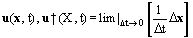Note that this defines two quantities: u(x, t) is the Eulerian specification of velocity and gives the spatial distribution of velocity as a function of time;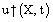is the Lagrangian specification of velocity and gives the velocity of material points of a fluid as a function of time. The Lagrangian specification is useful in certain contexts, for example, in flows of elasticoviscous Non-Newtonian Fluids in which the stress t in a material point X at time t depends on the flow in the neighbourhood of X at all times t* ≤ t. In general, however, use of a Lagrangian specification is cumbersome and an Eulerian specification is used instead.
Although a motion may be steady in an Eulerian sense, so that the velocity at each position is independent of time, a material point X of a body may accelerate. The acceleration a of X is defined by:
(2)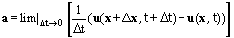Thus it follows that:
(3)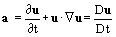where D/Dt denotes the substantial derivative.
It can be shown that:
(4)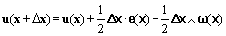where e denotes the strain-rate given by:
(5)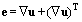and ω the vorticily given by:
(6)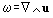Thus, the velocity field u(x + Δx) can be decomposed into three components: a translation   u(x), a straining (1/2) Δx · e(x) and a rotation - (1/2) Δx · ω(x).
An irrotational flow is one for which ω = Δ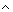u = 0. It can be shown that there exists a scalar potentialfor an irrotational flow such that:
(7)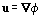Subject to some usually minor restrictions, an Inviscid Flow, that is flow of fluid of zero viscosity, which starts as an irrotational flow always remains an irrotational flow. For this reason, an inviscid flow is often analyzed using a scalar potential and called a potential flow. In contrast, a solenoidal flow is one for which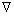·u = 0. It can then be shown that there exists a vector potential Ψ for a solenoidal flow such that:
(8)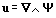Conservation of mass for an incompressible fluid can be shown to mean that the flow is solenoidal. For this reason, an incompressible flow is often analyzed using a vector potential. Furthermore, if the flow is two-dimensional, all but one component of Ψ vanish and u can be expressed in terms of a Streamfunction ψ. Thus, if u is two-dimensional and planar, that is in an appropriate rectangular or Cartesian coordinate system it only has an x-component and a y-component but no z-component, Ψ = ψiz and hence:
(9)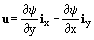where ixiy and iz denote unit vectors in the x-direction, y-direction and z-direction, respectively. The streamfunction y is constant along a Streamline, which is a line instantaneously at every point aligned with the local flow direction. As a result, streamlines are close together in those parts of a flow which are moving fast and far apart in those which are moving slowly.
There are usually two kinds of forces acting on a fluid:
• long-range forces, such as gravitational forces, which generally vary only slowly if at all over the fluid;
• short-range forces, such as viscous forces, which have a molecular origin and are, as a result, generally negligible unless there is physical contact between parts of the fluid. Long-range forces are called body forces or volume forces. Short-range forces can be approximated by forces on the surface of each part of the fluid: such surface forces lead to the concept of stressτ in a fluid, and in particular to components called Shear Stress and Normal Stress.
It is assumed that mass, linear momentum, angular momentum and energy are conserved. The conservation equations resulting from these Conservation Laws are:
(10)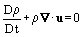(11)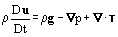(12)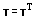(13)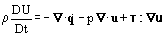where ρ denotes density, u velocity, g gravitational acceleration, p pressure, τ stress, U specific internal energy,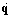heat flux and t time. The mass conservation equation (10) is often referred to as the continuity equation. The linear momentum equation (11) is often referred to as the equation of motion. The angular momentum conservation equation (12) is in a form appropriate to a nonpolar fluid, that is a fluid for which the angular momentum is just the moment of the linear momentum. The energy conservation equation (13) is appropriate to a system in which there is no heat generation by chemical or nuclear reaction and is an expression of the first law of thermodynamics.
Note that, for incompressible fluids, that is fluids of constant density ρ, p is not the thermodynamic pressure, which is in fact undefined for such fluids. Furthermore, the gravitational acceleration g can be eliminated from (11) by defining a modified pressure P:
(14)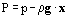where x denotes spatial position. Then (11) becomes:
(15)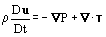In certain problems such as those involving free surfaces, however, pressure appears explicitly in the boundary conditions and gravity cannot, therefore, be eliminated. If the specific internal energy U is independent of the kinematics of the flow, then classical thermodynamic arguments can be applied. It can then be shown that (13) becomes:
(16)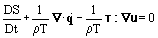where S denotes specific entropy and T absolute temperature. The term (1/ρT) τ·u is called the irreversible dissipation. It can be shown using the second law of thermodynamics:
(17)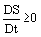that, for an incompressible fluid:
(18)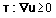that is the irreversible dissipation is nonnegative.
The conservation equations (10), (11), (12) and (13) govern the flow of any fluid. They describe how the density ρ, velocity u and specific internal energy U vary for a flowing fluid. They do this, however, by reference to the otherwise unknown pressure p, heat fluxand stress τ (though conservation of angular momentum does interrelate some components of τ). Equations are clearly needed for p,and τ if well-posed problems are to be formulated. These equations must be obtained by phenomenological and molecular arguments corroborated by experiments. The equations will, in general, differ for different materials. Indeed, it is in these equations that the nature of a given material is manifested. The equations are called constitutive equations or equations of state because they describe the constitution or state of a material. Perhaps the most commonly used constitutive equations are those for:
• an incompressible fluid, that is one for which density ρ is constant so that (10) becomes:
(19)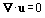• Newtonian fluid for which stress τ is related to strain-rate e given by (5) thus:
(20)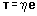where η denotes viscosity: then (11) becomes:
(21)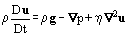which are called the Navier-Stokes equations;
• Fourier fluid for which heat fluxis related to temperature T thus:
(22)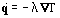where λ denotes thermal conductivity then for a Newtonian fluid (13) becomes:
(23)where c denotes specific heat.
Other constitutive equations exist: for an inviscid fluid, η = 0 and so τ = 0; for a Non-Newtonian Fluid τ is given by a rather more complex equation than (20).
The combined conservation-constitutive equations (10) or (19), (21) and (23) determine the behavior of a Newtonian Fourier fluid within a flow domain. In order to determine the flow fully, however, additional conditions must be specified. Initial conditions are required on ρ, u and T in order that the equations can be solved. Specification of initial conditions usually poses few problems in practice. Indeed, no initial conditions are needed if the flow is steady in an Eulerian sense, that is if ∂ρ/∂t = 0, ∂u/∂t = 0 and ∂T/∂t = 0. Because the conservation-constitutive equations apply within but not at the edge of the flow domain, boundary conditions are also needed to determine ρ, u and T completely. At a rigid, impermeable wall, the normal component of the fluid velocity u at the wall equals the normal component of the wall velocity uw:
(24)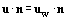where n denotes the unit normal to the fluid at the wall. If the wall is at rest in the reference frame being used, then u·n vanishes at the wall. Boundary condition (24) is referred to as the no flow-through condition. If the fluid is inviscid, then the fluid can slip over the wall and the tangential components of u cannot be specified. If, on the other hand, the fluid is viscous, for example, it is Newtonian, then molecular arguments corroborated by experiments show that the tangential components of u at the wall equal the tangential components of uw, so that there is no slip at the wall:
(25)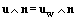Combining (24) and (25) yields:
(26)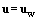Boundary condition (26) is referred to as the no slip condition. In distinction to (24), which is entirely kinematic in origin, (25) is dynamic in origin so that (26) is partly kinematic and partly dynamic in origin. Arguments for the validity of the no slip condition are molecular and empirical in nature. Molecular arguments are based on notions of thermodynamic equilibrium at a wall and hence equality of the velocities of the molecules of the fluid and those of the wall. Empirical arguments are based on the extensive experimental corroboration of the consequences of assuming that there is no slip at a wall.
Boundary conditions on temperature are based on two notions. The first is that the heat flux must be continuous normal to the wall, in order that energy is conserved. Thus, for a Fourier fluid and a Fourier wall:
(27)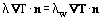where λw denotes the thermal conductivity of the wall. The second notion is that of thermodynamic equilibrium, which implies that the fluid temperature T at the wall is the same as the wall temperature Tw:
(28)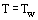It appears to be impossible to develop general methods for obtaining exact solutions of equations (10) or (19), (21) and (23) subject to appropriate boundary and initial conditions. There is, therefore, a need for a systematic method for solving them approximately, either analytically or numerically using computers. A description of the methods involved in the latter, called Computational Fluid Dynamics, is beyond the scope of this article [instead see Roache (1972) and Crochet, Davies and Walters (1984)]. A rational basis exists for the development of analytical methods by the use of order-of-magnitude arguments based on dimensionless formulations. Most flows admit a characteristic length Lc, a characteristic speed Uc and a characteristic temperature Tc. Thus, for example, for flow in a long narrow pipe, Lc might be the pipe radius, Uc the mean fluid velocity along the pipe and Tc the temperature of the pipe wall. Define dimensionless variables (distinguished by a superscript asterisk) as follows: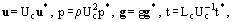(29)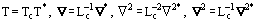where g = |g|. Then for an incompressible fluid (21) and (23) become respectively:
(30)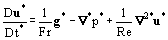(31)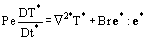where:
• the Froude NumberFr, is given by:
(32)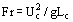which may be interpreted as the ratio of inertial forces to gravitational forces;
• the Reynolds NumberRe, is given by:
(33)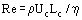which may be interpreted as the ratio of inertial forces to viscous forces;
• the Peclet NumberPe, is given by:
(34)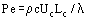which may be interpreted as the ratio of convection to conduction;
• the Brinkman numberBr, is given by:
(35)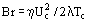which may be interpreted as the ratio of heat generation by viscous dissipation to conduction (note that the factor of two in (35) is entirely arbitrary).
There are two main reasons for making (21) and (23) dimensionless. The first is as follows. Suppose that two flow problems have the same relative geometry, that is they are geometrically similar (an example is flow in two pipes of different size but of the same length-to-radius ratio), the same dimensionless boundary and initial conditions and the same values of the dimensionless groups FrRePe and Br. Then the dimensionless solutions of the two problems are identical and the flows are said to be dynamically similar. The second reason concerns making rational simplifications to the flow equations based on order-of-magnitude arguments, in order to obtain approximate solutions. To see this, consider simplifications to the linear momentum conservation equation, that is the dimensionless Navier-Stokes equations (30). What generally matters is the relative importance of the inertial term Du*/Dt* and the viscous term 1/Re2*u*, in which the magnitude of the Reynolds number Re plays a central role.
If Re is very small, that is if Re << 1, it follows from (30) that the inertial term should be negligible compared with the viscous term and hence that the Navier-Stokes equations should become approximately:
(36)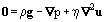These are Stokes' equations. If for a given flow Re is low enough that inertial effects can be neglected, that flow is called a slow flow, a creeping flow or a Stokes flow. (An interesting feature of solutions of Stokes' equations is that, if an arbitrary cylindrical cross-section is projected normally from one flat wall to another parallel flat wall, the streamlines of the flow about the cylinder are identical to those for inviscid potential flow about the same cylinder: this is called Hele-Shaw flow.) For steady flow at speed U round a stationary solid sphere of radius R in an unbounded incompressible Newtonian fluid of density ρ and viscosity η, it can be shown that the magnitude F of the force exerted on the sphere is given by:
(37)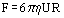provided the flow is slow, that is provided:
(38)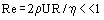The expression for F in (37) is called Stokes' Law experimentally, it is found to be valid for Re < 0.1 or so. In dimensionless form, Stokes' law is:
(39)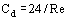where the drag coefficient, Cd, which is a dimensionless drag force, is given by:
(40)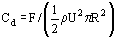It can be shown that the analogous steady flow past a long stationary solid (right circular) cylinder in an unbounded incompressible Newtonian fluid does not exist. This is referred to as Stokes' paradox. Of course, it is not a real paradox, since it is clearly possible for fluid to flow past a cylinder at arbitrarily small Re: it merely means that the problem has been over-constrained by making at least one assumption too many.
If Re is very large, that is if Re >> 1, it follows from (30) that the viscous term should be negligible compared with the inertial term and hence that the Navier-Stokes equations should become approximately:
(41)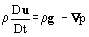These are Euler's equations which govern the flow of an inviscid fluid. For a steady incompressible inviscid flow, it can be shown from (41) dial:
(42)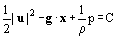where x denotes position and C a constant. This is Bernoulli's Equation: it may be interpreted physically as stating that, for steady flow of an incompressible inviscid fluid, the sum of the kinetic energy per unit mass (1/2 |u|2), the potential energy per unit mass (−g·x) and the pressure energy per unit mass (1/ρ·p) is constant. Equivalent, if more complicated, forms of Bernoulli's equation apply for an unsteady or compressible flow. For steady inviscid flow round a stationary solid sphere in an unbounded incompressible Newtonian fluid, it can be shown that the magnitude F of the force exerted on the sphere is given by:
(43)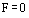Thus, an incompressible inviscid fluid moving at constant velocity round a stationary sphere exerts no drag force on the sphere. This is referred to as d'Alembert's paradox. Experimental evidence shows that, for flow of a real fluid, the viscosity of which never vanishes, (43) is false, no matter how large Re may be. The reason for this is that Re >> 1 implies that flows are effectively inviscid and hence approximately irrotational almost, but not quite, everywhere. Viscous effects, which lead to drag forces, are always important near and downstream of walls in Boundary Layers and wakes, respectively.
If Re is neither very high nor very low, that is if Re ~ 1, viscous and inertial forces should both be comparable and hence both be significant. Provided Re is not very large, there will be Laminar Flow: if Re is very large, there will be Turbulent Flow. Two main simplifications are possible if the flow is laminar. One is when there is fully-developed flow. The other is when there is boundary layer flow.
It happens that, in some common laminar flows, the presence of walls means that the acceleration term ρ Du/Dt in (21) is negligible even though Re is not small: such a flow is said to be locally fully-developed, or fully-developed if ρ Duu/Dt = 0. A locally fully-developed flow is governed by Stokes' equations (36). Laminar Channel Flow is fully-developed except near the inlet and outlet, provided the channel is sufficiently long. Poiseuille Flow is pressure-induced channel flow in a long duct, usually a pipe. The volumetric flow rate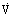of a fluid of viscosity η through a pipe of radius R and length L is related to the pressure drop Δp by:
(44)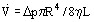This is the Hagen-Poiseuille equation. Pressure-driven flow is to be distinguished from drag-induced flow such as Couette Flow between two parallel flat plates a constant distance apart: one plate remains stationary, the other moves in its own plane. Provided the plates are long compared with the distance between them, the flow is fully-developed almost everywhere. If an incompressible, Newtonian liquid of density ρ and viscosity η falls in a layer of thickness h on a flat vertical plate of infinite extent, the liquid flows down the plate as a result of the gravitational acceleration. Beside the liquid is a gas of negligible density and viscosity and uniform pressure. Analysis of such Falling Film Flow shows that the volumetric flow rateper unit width is given by:
(45)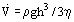A high Re flow is effectively inviscid almost everywhere. Near a wall, however, in what is called a boundary layer, viscous effects are always important. The reason for this is that there is no slip of a viscous fluid at a rigid impermeable wall, however small its viscosity may be. Analysis of such high Re flows is based on the assumption that there are two distinct regions in the flow:
• a region away from the walls in which viscous effects are negligible;
• a thin region immediately adjacent to the walls in which viscous effects are not negligible.
In the region away from the walls, the Navier-Stokes equations simplify to Euler's equations. In the boundary layer region adjacent to the walls, inertial effects and viscous effects are both significant: because it is thin, however, the transverse velocity is much smaller than the axial velocity, axial derivatives of velocity components are much smaller than transverse derivatives of those same components and the transverse pressure gradient is much smaller than the axial pressure gradient. For flow at velocity U past a semi-infinite flat plate, it can be shown that, if the axial velocity ux = ∂ψ/∂y = Ufβ, where:
(46)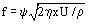(47)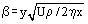(see Figure 1) then f satisfies Blasius' equation:
(48)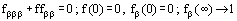where a subscript denotes differentiation. The solution, obtained numerically, is shown in Figure 2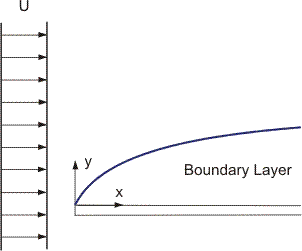Figure 1. Flow past flat plate.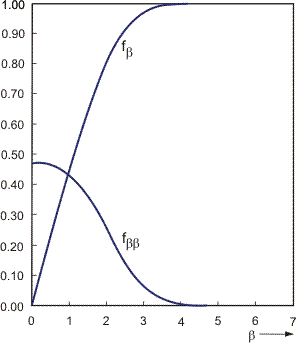Figure 2. Solution of Blasius' equation.
Any laminar flow becomes unstable if Re or some other equivalent dimensionless number becomes sufficiently large. The initial instability is usually a Secondary Flow superimposed on the basic laminar flow. When Re is sufficiently large, the flow becomes turbulent. A Turbulent Flow is always a high Re flow. Two main classes of turbulent flow must be distinguished:
• a wall flow, for example, flow in a pipe;
• a free or External Flow, for example, flow of a jet.
The details of the transition from laminar to turbulent flow are not well understood. In a turbulent flow, the flow variables such as velocity u and pressure p fluctuate in space and time in an apparently random manner. The fluctuations, which are often called eddies, are, as far as is known, completely predictable from the conservation equations, given appropriate boundary and initial conditions. Furthermore, it appears that many turbulent flows are dominated by large-scale motions which are not random. Because of the apparent randomness of turbulent flows, statistical flow descriptions are appropriate and average value of a flow quantity such as u or p are used. A volume average cannot be used, however, because a turbulent flow comprises fluctuations over a range of length-scales. Similarly, a time average cannot be used because a turbulent flow can be unsteady on average. Instead, an ensemble average is used in which an average is taken over many repetitions of an apparently identical flow, that is one with as nearly the same boundary and initial conditions as possible. For a stationary turbulent flow, that is a turbulent flow which is steady on average, the ergodic hypothesis is that, under certain fairly general conditions, the ensemble average of each flow variable is the same as its time average. For such a flow, a time average can be used instead of an ensemble average: indeed such time averages are what are commonly used in experimental measurements. Decomposition of each flow variable into an ensemble average component (denoted by an overbar) and a fluctuating component (denoted by a prime) permits definition of the relative turbulent intensity Ir given by:
(49)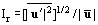In a typical turbulent flow, Ir ~ 0.1.
The mass and linear momentum conservation equations (19) and (21) for an incompressible Newtonian fluid can be averaged to give:
(50)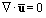(51)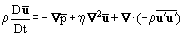which are Reynolds' equations. Note that (50) for ū is the same as (19) for u but (51) for ū anddiffers from (21) for u and p: there is an extra term involving the so-called Reynolds' stress—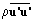in (51). Its presence in (51) illustrates the closure problem in turbulence; there are more unknowns than equations—a closure problem always arises when a nonlinear system is averaged since averaging loses information. In order to eliminate the closure problem in turbulence, a turbulence model is needed for the Reynolds stress. Such a model cannot, however, be obtained from the flow equations: it must be obtained using other arguments.
There are many general texts on fluid mechanics. Four excellent ones are Batchelor (1967), Denn (1980), Landau and Lifshitz (1959) and Streeter (1961); another is Richardson (1989). A good understanding of fluid mechanics is greatly enhanced by observing real flows, that is, by flow visualization. An outstanding collection of photographs is to be found in Van Dyke (1982). Many more are to be found in Tritton (1977). Good introductions to the mathematics underlying fluid mechanics are given in Leigh (1968) and Truesdell (1977).
It is as yet impossible to prove whether or not the flow equations for an incompressible Newtonian fluid have a solution and, if so, whether the solution is unique for arbitrary initial and boundary conditions. The standard text on this subject is Ladyzhenskaya (1969). A more recent one is Temam (1977); a rather more readable account is in Shinbrot (1973). The existence and uniqueness of a solution of the flow equations do not guarantee that the flow corresponding to the solution can actually occur in practice: the flow must also be stable. The stability of flows is discussed in Chandrasekhar (1961) and Drazin and Reid (1981).
A comprehensive treatment of slow flow is to be found in Happel and Brenner (1973). An excellent text, with particularly good coverage of the paradoxes which arise in slow and inviscid flow is Van Dyke (1964). The standard text on inviscid flow is Lamb (1932). A very good, more recent, one is Lighthill (1986). A masterly discussion of wave motions in fluids is to be found in Lighthill (1978). A very readable account of locally fully-developed flow and boundary layer flow is given in White (1974). The standard text on boundary layer flow is Schlichting (1968); an extremely comprehensive one is Rosenhead (1963). An excellent introductory text on turbulence is Tennekes and Lumley (1972), while more advanced ones are Bradshaw (1976) and Hinze (1959). Turbulence models are discussed in Launder and Spalding (1972).
A good and comprehensive text on incompressible flows is Panton (1984). A standard and excellent text on compressible flow is Courant and Friedrichs (1948). A very useful introduction to one-dimensional compressible flow, for example in ducts, is given by Daneshyar (1976).
A good text on the mechanics of isolated bubbles, drops and particles is Clift, Grace and Weber (1978). A comprehensive text on Multiphase Flow is Hetsroni (1982), while a standard account of multiphase flows and many related topics is Levich (1962).